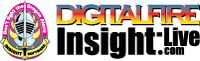•The secret to cool bodies and glazes is a lot of testing.
•The secret to know what to test is material and chemistry knowledge.
•The secret to learning from testing is documentation.
•The place to test, do the chemistry and document is an account at https://insight-live.com
•The place to get the knowledge is https://digitalfire.com

Sign-up at https://insight-live.com today.

# Grefco Fireclay

 Oxide Analysis Formula CaO 0.10% 0.006 MgO 0.50% 0.040 K2O 0.91% 0.031 Na2O 0.51% 0.026 TiO2 1.92% 0.077 Al2O3 31.81% 1.000 SiO2 52.11% 2.780 Fe2O3 1.42% 0.028 LOI 10.71 Oxide Weight 286.28 Formula Weight 320.62

## XML for Import into INSIGHT

<?xml version="1.0" encoding="UTF-8"?> <material name="Grefco Fireclay" descrip="" searchkey="" loi="10.71" casnumber="70694-09-6"> <oxides> <oxide symbol="CaO" name="Calcium Oxide, Calcia" status="" percent="0.100" tolerance=""/> <oxide symbol="MgO" name="Magnesium Oxide, Magnesia" status="" percent="0.500" tolerance=""/> <oxide symbol="K2O" name="Potassium Oxide" status="" percent="0.910" tolerance=""/> <oxide symbol="Na2O" name="Sodium Oxide, Soda" status="" percent="0.510" tolerance=""/> <oxide symbol="TiO2" name="Titanium Dioxide, Titania" status="" percent="1.920" tolerance=""/> <oxide symbol="Al2O3" name="Aluminum Oxide, Alumina" status="U" percent="31.810" tolerance=""/> <oxide symbol="SiO2" name="Silicon Dioxide, Silica" status="" percent="52.110" tolerance=""/> <oxide symbol="Fe2O3" name="Iron Oxide, Ferric Oxide" status="" percent="1.420" tolerance=""/> </oxides> <volatiles> <volatile symbol="LOI" name="Loss on Ignition" percent="10.710" tolerance=""/> </volatiles> </material>